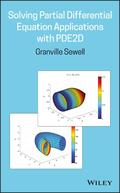John Wiley & Sons Solving Partial Differential Equation Applications with PDE2DSolve engineering and scientific partial differential equation applications using the PDE2D software.. Product #: 978-1-119-50793-2 Regular price: \$85.89 \$85.89 Auf Lager

# Solving Partial Differential Equation Applications with PDE2D1. Auflage Dezember 2018
224 Seiten, Hardcover
Wiley & Sons Ltd

ISBN: 978-1-119-50793-2
John Wiley & Sons

Jetzt kaufen

### Preis: 91,90 €

Preis inkl. MwSt, zzgl. Versand

Solve engineering and scientific partial differential equation applications using the PDE2D software developed by the author

Solving Partial Differential Equation Applications with PDE2D derives and solves a range of ordinary and partial differential equation (PDE) applications. This book describes an easy-to-use, general purpose, and time-tested PDE solver developed by the author that can be applied to a wide variety of science and engineering problems. The equations studied include many time-dependent, steady-state and eigenvalue applications such as diffusion, heat conduction and convection, image processing, math finance, fluid flow, and elasticity and quantum mechanics, in one, two, and three space dimensions.

The author begins with some simple "0D" problems that give the reader an opportunity to become familiar with PDE2D before proceeding to more difficult problems. The book ends with the solution of a very difficult nonlinear problem, which requires a moving adaptive grid because the solution has sharp, moving peaks. This important book:
* Describes a finite-element program, PDE2D, developed by the author over the course of 40 years
* Derives the ordinary and partial differential equations, with appropriate initial and boundary conditions, for a wide variety of applications
* Offers free access to the Windows version of the PDE2D software through the author's website at www.pde2d.com
* Offers free access to the Linux and MacOSX versions of the PDE2D software also, for instructors who adopt the book for their course and contact the author at www.pde2d.com

Written for graduate applied mathematics or computational science classes, Solving Partial Differential Equation Applications with PDE2D offers students the opportunity to actually solve interesting engineering and scientific applications using the accessible PDE2D.

Preface vii

I Introduction to PDE2D 1

I.1 The Collocation and Galerkin Finite Element Methods 1

I.2 The PDE2D User Interfaces 7

I.3 Accuracy 11

I.4 Computer Time and Memory 13

I.5 Programming Hints 17

1 The Damped Spring and Pendulum Problems 21

1.1 Derivation of the Damped Spring and Pendulum Equations 21

1.2 Damped Spring and Pendulum Examples 23

1.3 Problems 24

2 Beam and Plate Bending 31

2.1 Derivation of Beam Bending Equation 31

2.2 Derivation of Plate Bending Equation 32

2.3 Beam and Plate Examples 33

2.4 Problems 34

3 Diffusion and Heat Conduction 39

3.1 Derivation of Diffusion Equation 39

3.2 Diffusion and Heat Conduction Examples 40

3.3 Problems 51

4 Pricing Options 61

4.1 Derivation of Black-Scholes Equation 61

4.2 Option Pricing Examples 65

4.3 Problems 70

5 Elasticity 75

5.1 Derivation of Elasticity Equations 75

5.2 Elasticity Examples 77

5.3 Problems 81

6 Incompressible Fluid Flow 95

6.1 Derivation of Navier-Stokes Equations 95

6.2 Stream Function and PenaltyMethod Approaches 97

6.3 Fluid Flow Examples 97

6.4 Problems 105

7 The Schrödinger and Other Eigenvalue Equations 119

7.1 The Schrödinger Equation 119

7.2 Schrödinger and Maxwell Equations Examples 119

7.3 Problems 126

8 Minimal Surface and MembraneWave Equations 137

8.1 Derivation of Minimal Surface Equation 137

8.2 Derivation of MembraneWave Equation 138

8.3 Examples 140

8.4 Problems 142

9 The KPIWave Equation 149

9.1 A Difficult Nonlinear Problem 149

9.2 Numerical Results 155

Appendix A: Formulas from Multivariate Calculus 161

Appendix B: Algorithms Used by PDE2D 163

Appendix C: Equations Solved by PDE2D 183

Appendix D: Problem 5.7 Local Solvers 193

References 205

Index 207
Granville Sewell, PhD, is Professor in the Mathematics department at the University of Texas-El Paso, El Paso, TX. Dr. Sewell is the author of The Numerical Solution of Ordinary and Partial Differential Equations, Second Edition, and Computational Methods of Linear Algebra, Second Edition, both published by Wiley.

G. Sewell, University of Texas at El Paso